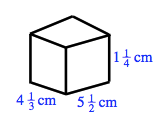### Home > CC1MN > Chapter 9 Unit 9 > Lesson CC1: 9.2.3 > Problem9-61

9-61.

Find the volume and surface area of a box with dimensions of $5\frac{1}{2}$ cm by $4\frac{1}{3}$ cm by $1\frac{1}{4}$ cm.$\text{Volume}\ =\ 29\frac{19}{24}\;\text{cm}^3$
$\text{Surface Area}\ =\ 72\frac{1}{4}\;\text{cm}^2$